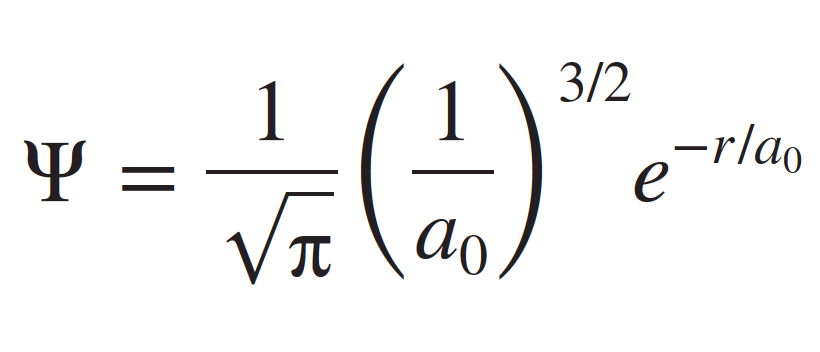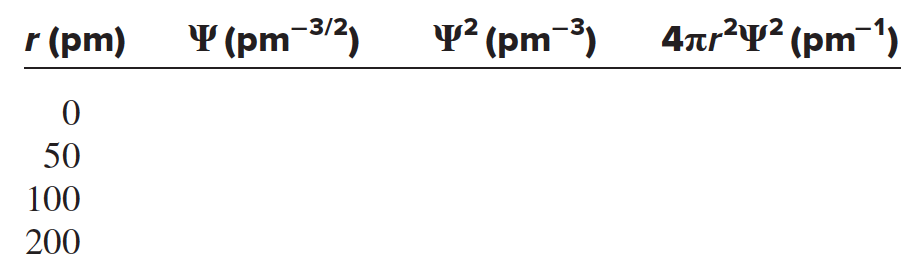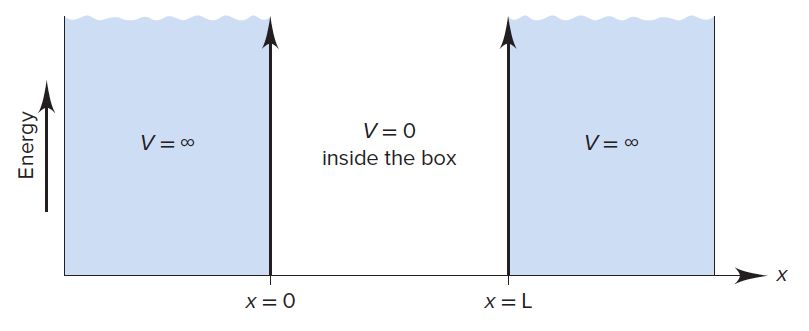# Problem: The quantum-mechanical treatment of the hydrogen atom gives this expression for the wave function, ψ, of the 1s orbital:where r is the distance from the nucleus and a0 is 52.92 pm. The probability of finding the electron in a tiny volume at distance r from the nucleus is proportional to ψ2. The total probability of finding the electron at all points at distance r from the nucleus is proportional to 4πr2ψ2. Calculate the values (to three significant figures) of ψ, ψ2, and 4πr2ψ2 to fill in the following table and sketch a plot of each set of values versus r. Compare the latter two plots with those in the figure below.

⚠️Our tutors found the solution shown to be helpful for the problem you're searching for. We don't have the exact solution yet.

###### Problem Details

The quantum-mechanical treatment of the hydrogen atom gives this expression for the wave function, ψ, of the 1s orbital:where r is the distance from the nucleus and a0 is 52.92 pm. The probability of finding the electron in a tiny volume at distance r from the nucleus is proportional to ψ2. The total probability of finding the electron at all points at distance r from the nucleus is proportional to 4πr2ψ2. Calculate the values (to three significant figures) of ψ, ψ2, and 4πr2ψ2 to fill in the following table and sketch a plot of each set of values versus r. Compare the latter two plots with those in the figure below.